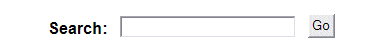Hidden Squares Puzzle Activity

Easier Hidden Squares Puzzles

All Column and Row Totals Given
5 by 5 easy puzzle (25% of squares colored-in)
6 by 6 easy puzzle (25% of squares colored-in)
7 by 7 easy puzzle (25% of squares colored-in)
8 by 8 easy puzzle (25% of squares colored-in)
9 by 9 easy puzzle (25% of squares colored-in)
10 by 10 easy puzzle (25% of squares colored-in)
11 by 11 easy puzzle (25% of squares colored-in)
12 by 12 easy puzzle (25% of squares colored-in)
13 by 13 easy puzzle (25% of squares colored-in)
14 by 14 easy puzzle (25% of squares colored-in)
15 by 15 easy puzzle (25% of squares colored-in)

Half of the Column and Row Totals Given
5 by 5 easy puzzle (25% of squares colored-in)
6 by 6 easy puzzle (25% of squares colored-in)
7 by 7 easy puzzle (25% of squares colored-in)
8 by 8 easy puzzle (25% of squares colored-in)
9 by 9 easy puzzle (25% of squares colored-in)
10 by 10 easy puzzle (25% of squares colored-in)
11 by 11 easy puzzle (25% of squares colored-in)
12 by 12 easy puzzle (25% of squares colored-in)
13 by 13 easy puzzle (25% of squares colored-in)
14 by 14 easy puzzle (25% of squares colored-in)
15 by 15 easy puzzle (25% of squares colored-in)

No Column and Row Totals Given
5 by 5 easy puzzle (25% of squares colored-in)
6 by 6 easy puzzle (25% of squares colored-in)
7 by 7 easy puzzle (25% of squares colored-in)
8 by 8 easy puzzle (25% of squares colored-in)
9 by 9 easy puzzle (25% of squares colored-in)
10 by 10 easy puzzle (25% of squares colored-in)
11 by 11 easy puzzle (25% of squares colored-in)
12 by 12 easy puzzle (25% of squares colored-in)
13 by 13 easy puzzle (25% of squares colored-in)
14 by 14 easy puzzle (25% of squares colored-in)
15 by 15 easy puzzle (25% of squares colored-in)

Blank Hidden Squares Puzzles
5 by 5 blank puzzle
6 by 6 blank puzzle
7 by 7 blank puzzle
8 by 8 blank puzzle
9 by 9 blank puzzle
10 by 10 blank puzzle
11 by 11 blank puzzle
12 by 12 blank puzzle
13 by 13 blank puzzle
14 by 14 blank puzzle
15 by 15 blank puzzle

More Challenging Hidden Squares Puzzles
5 by 5 puzzle - more difficult to solve
6 by 6 puzzle - more difficult to solve
7 by 7 puzzle - more difficult to solve
8 by 8 puzzle - more difficult to solve
9 by 9 puzzle - more difficult to solve
10 by 10 puzzle - more difficult to solve
11 by 11 puzzle - more difficult to solve
12 by 12 puzzle - more difficult to solve
13 by 13 puzzle - more difficult to solve
14 by 14 puzzle - more difficult to solve
15 by 15 puzzle - more difficult to solve

>Greater> <Less< Than Puzzle

Easier - >Greater> <Less< Than Puzzles - Some extra numbers given

Easier: Positive Numbers
5 by 5 easy puzzle (2 extra numbers given)
6 by 6 easy puzzle (3 extra numbers given)
7 by 7 easy puzzle (3 extra numbers given)
8 by 8 easy puzzle (4 extra numbers given)
9 by 9 easy puzzle (4 extra numbers given)
10 by 10 easy puzzle (5 extra numbers given)

Easier: Negative Numbers
5 by 5 easy negative number puzzle (2 extra numbers given)
6 by 6 easy negative number puzzle (3 extra numbers given)
7 by 7 easy negative number puzzle (3 extra numbers given)
8 by 8 easy negative number puzzle (4 extra numbers given)
9 by 9 easy negative number puzzle (4 extra numbers given)

>Greater> <Less< Than Puzzles

Positive Numbers
5 by 5 puzzle
6 by 6 puzzle
7 by 7 puzzle
8 by 8 puzzle
9 by 9 puzzle
10 by 10 puzzle

Negative Numbers
5 by 5 negative number puzzle
6 by 6 negative number puzzle
7 by 7 negative number puzzle
8 by 8 negative number puzzle
9 by 9 negative number puzzle

>Greater> <Less< Than Puzzles - Students may use any number from 1 to 9

Positive Numbers - Using all numbers 1 to 9
5 by 5 puzzle (using numbers 1 to 9)
6 by 6 puzzle (using numbers 1 to 9)
7 by 7 puzzle (using numbers 1 to 9)
8 by 8 puzzle (using numbers 1 to 9)

Negative Numbers - Using all numbers -1 to -9
5 by 5 negative number puzzle (using all numbers -1 to -9)
6 by 6 negative number puzzle (using all numbers -1 to -9)
7 by 7 negative number puzzle (using all numbers -1 to -9)
8 by 8 negative number puzzle (using all numbers -1 to -9)

Visit edHelper.comReading and Writing   Reading Comprehension   Read and Color Books   Literature Units   Writing  PreK-3   Kindergarten   Phonics   PreK-3 Themes  Language   Language Arts   Spelling   Vocabulary   Enter Word Lists  Special Education   Special Education  Foreign Languages   Foreign Languages   Spanish   French   German   Italian Math   Math   Middle School Math   Algebra  Critical Thinking   Critical Thinking  Puzzles   Make Puzzles   Quick Prints  Social Studies   Social Studies   Geography   United States  Science   Science   Health   Animals  More   High School   Test Prep   Art   Classroom Helpers   Monthly Themes   Daily Skills Review

If you prefer not to receive news from edHelper:  Unsubscribe from the edHelper newsletter